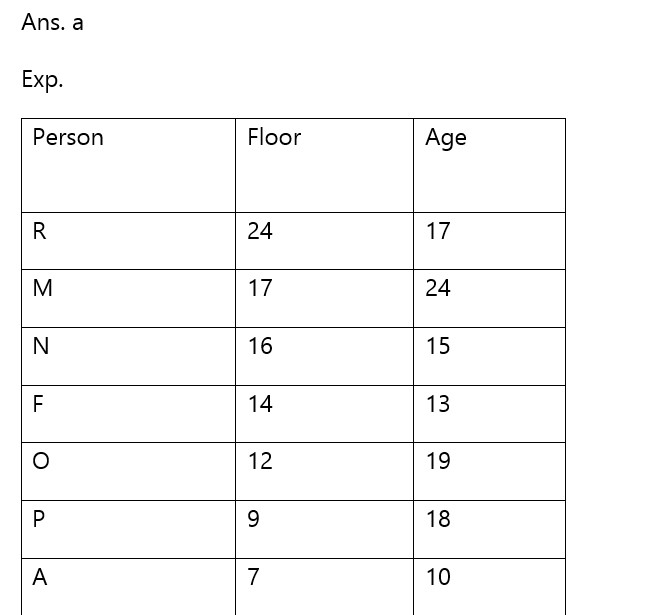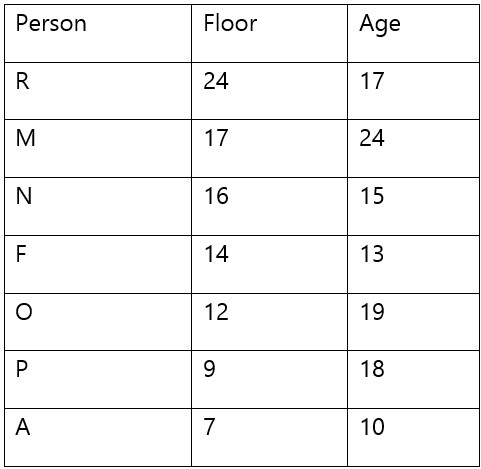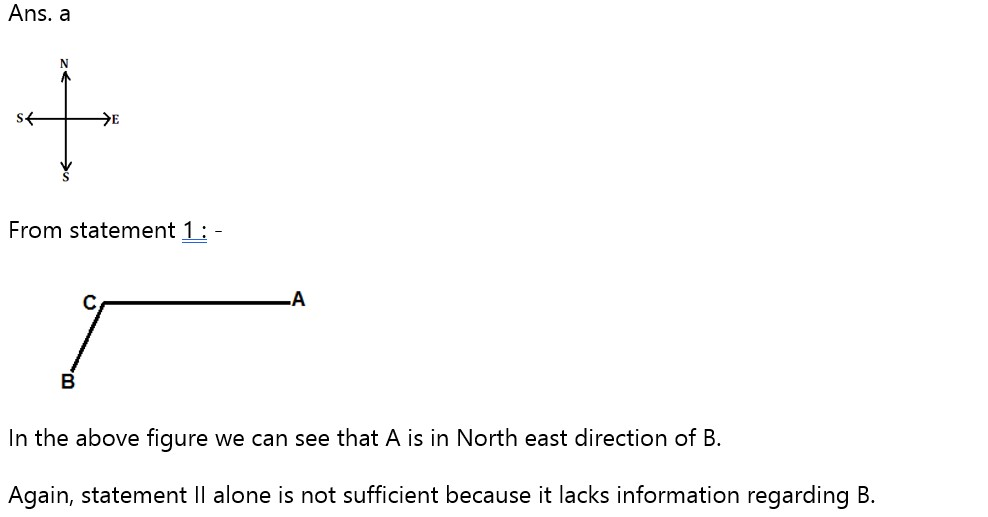# SBI PO Prelims Reasoning Ability Quiz – 36

## SBI PO Prelims Reasoning Ability Quiz

Aspirants have a strong possibility of scoring well in the Reasoning Ability section if they practice quality questions on a regular basis. This section takes the least amount of time if the practice is done every day in a dedicated manner. In this article, we have come up with the SBI PO Prelims Reasoning Ability Quiz to help you prepare better. Candidates will be provided with a detailed solution for each question in this SBI PO Prelims Reasoning Ability Quiz. This SBI PO Prelims Reasoning Ability Quiz includes a variety of questions ranging in difficulty from easy to tough. This SBI PO Prelims Reasoning Ability Quiz is totally FREE. This SBI PO Prelims Reasoning Ability Quiz has important Reasoning Ability Questions and Answers that will help you improve your exam score. Aspirants must practice this SBI PO Prelims Reasoning Ability Quiz in order to be able to answer questions quickly and efficiently in upcoming exams.

Directions (1-5): Read the following information carefully and answer the questions given below.

There are seven persons A, R, M, N, O, F and P living in a building with twenty-four floors. Bottom most floor is numbered as first floor while the topmost floored being numbered as twenty fourth. No two persons live on the same floor. They live on floor number 24, 17, 16, 14, 12, 9 and 7 but not necessarily in the same order. Rest of the floors are empty. These seven persons have different ages viz. 24, 17, 19, 18, 15, 13 and 10 but not necessarily in the same order. The one who is seventeen years old does not live on an odd numbered floor. Only two persons live below O’s floor. No one is older than M who has six empty floors above his floor. Difference between the ages of R and O is less than four years. R has more than ten empty floors below his floor. Only two persons live above N. P lives on one of the floors above A. Difference between the age and floor number of F is same as the difference between the age and floor number of N and that difference is not an even number. O is as much older than P as R is younger than P. F is not the youngest.

1. How many empty floors are there between the floors in which the youngest and the oldest person are living?

(a) Five

(b) Six

(c) Three

(d) Eleven

(e) None of these1. What is the sum of the ages of M, N, F and P?

(a) Less than fifty

(b) Sixty Five

(c) Fifty Six

(d) Seventy

(e) None of these

Ans. d

Exp.1. How many persons are living between the floors of P and A?

(a) Two

(b) Three

(c) Five

(d) No one

(e) None of these

Ans. d

Exp.1. How many persons are living above P’s floor, who are younger than him?

(a) Three

(b) Four

(c) Two

(d) One

(e) None of these

Ans. a

Exp.1. The youngest amongst them is living on which of the following floors?

(a) Seventh

(b) Twenty fourth

(c) Ninth

(d) Twelfth

(e) None of these

Ans. a

Exp.Directions (6-10): Each of the questions below consists of a question and two statements numbered I and II given below it. You have to decide whether the data provided in the statements are sufficient to answer the question. Read both the statements and give answer.

1. How is ‘sunday’ written in a code language?
I.
‘January march sunday april monday tuesday’ is written as ‘dd ee jj oo tt yy’ in that code language.
II.
‘June monday july april march tuesday’ is written as ‘dd ee ll oo ww yy’ in that code language.

(a) If the data in statement I alone are sufficient to answer the question, while the data in statement II alone are not sufficient to answer the question.

(b) If the data in statement II alone are sufficient to answer the question, while the data in statement I alone are not sufficient to answer the question.

(c) If the data either in statement I alone or in statement II alone are sufficient to answer the question.

(d) If the data in both statements I and II together are not sufficient to answer the question.

(e) If the data in both statements I and II together are necessary to answer the question.

Ans. d

Exp.  From both the statement we cannot find the code for ‘Sunday’.

1. A is in which direction of B?
I.
C is towards west of A and towards North-east of B.
II.
C is towards south of D, which is towards North-east of A.

(a) If the data in statement I alone are sufficient to answer the question, while the data in statement II alone are not sufficient to answer the question.

(b) If the data in statement II alone are sufficient to answer the question, while the data in statement I alone are not sufficient to answer the question.

(c) If the data either in statement I alone or in statement II alone are sufficient to answer the question.

(d) If the data in both statements I and II together are not sufficient to answer the question.

(e) If the data in both statements I and II together are necessary to answer the question.1. Amongst U, V and W, who scored the highest number of runs in the series XYZ? I. Total score made by U and W was more than V’s Score.
II. Number of total centuries and fifties made by V were higher than U and W both, in the series.

(a) If the data in statement I alone are sufficient to answer the question, while the data in statement II alone are not sufficient to answer the question.

(b) If the data in statement II alone are sufficient to answer the question, while the data in statement I alone are not sufficient to answer the question.

(c) If the data either in statement I alone or in statement II alone are sufficient to answer the question.

(d) If the data in both statements I and II together are not sufficient to answer the question.

(e) If the data in both statements I and II together are necessary to answer the question.

Ans. d

Exp.  We are not getting proper information from both the statements.

1. A, B, C, D, E and F are sitting in a circle, facing towards the centre of the circle. Who is sitting on the immediate left of A?
I. A is facing D. Only C is between A and B. F is between E and A.
II. F is on the immediate left of E. Only D is between B and E. C is not adjacent to F.

(a) If the data in statement I alone are sufficient to answer the question, while the data in statement II alone are not sufficient to answer the question.

(b) If the data in statement II alone are sufficient to answer the question, while the data in statement I alone are not sufficient to answer the question.

(c) If the data either in statement I alone or in statement II alone are sufficient to answer the question.

(d) If the data in both statements I and II together are not sufficient to answer the question.

(e) If the data in both statements I and II together are necessary to answer the question.

Ans. b

Exp.   From 2nd statement we can see that C is on the immediate left of A.

1. Amongst A, E, I, O and U, which book is having the least price?
I.Price of the book U is less than those of I and O only.
II.Price of A is less than O and more than E.

(a) If the data in statement I alone are sufficient to answer the question, while the data in statement II alone are not sufficient to answer the question.

(b) If the data in statement II alone are sufficient to answer the question, while the data in statement I alone are not sufficient to answer the question.

(c) If the data either in statement I alone or in statement II alone are sufficient to answer the question.

(d) If the data in both statements I and II together are not sufficient to answer the question.

(e) If the data in both statements I and II together are necessary to answer the question.

Ans. e

Exp.   I/O > O/I > U > A > E

So, E has least cost.

### Preparation Kit PDF

#### Most important PDF’s for Bank, SSC, Railway and Other Government Exam : Download PDF Now

AATMA-NIRBHAR Series- Static GK/Awareness Practice Ebook PDF Get PDF here
The Banking Awareness 500 MCQs E-book| Bilingual (Hindi + English) Get PDF here
AATMA-NIRBHAR Series- Banking Awareness Practice Ebook PDF Get PDF here
Computer Awareness Capsule 2.O Get PDF here
AATMA-NIRBHAR Series Quantitative Aptitude Topic-Wise PDF 2020 Get PDF here
Memory Based Puzzle E-book | 2016-19 Exams Covered Get PDF here
Caselet Data Interpretation 200 Questions Get PDF here
Puzzle & Seating Arrangement E-Book for BANK PO MAINS (Vol-1) Get PDF here
3